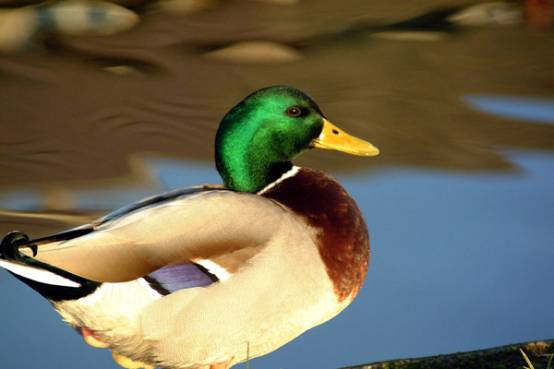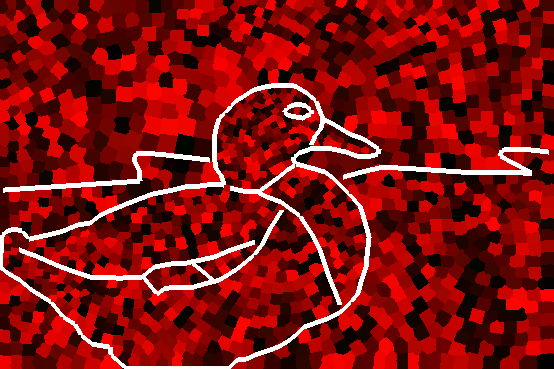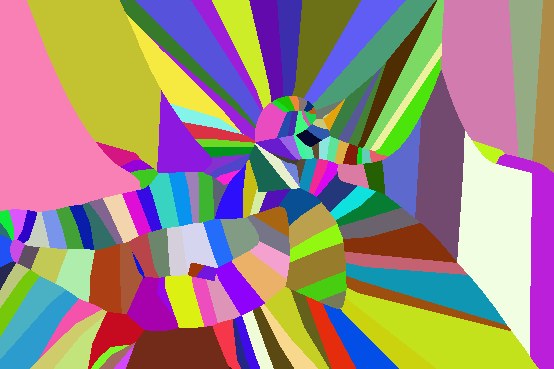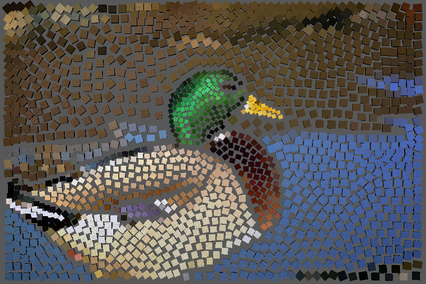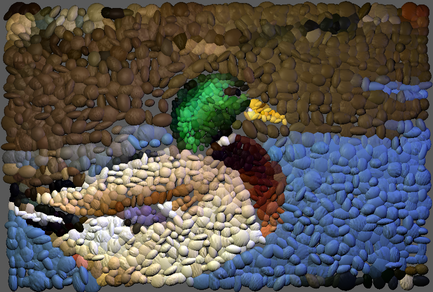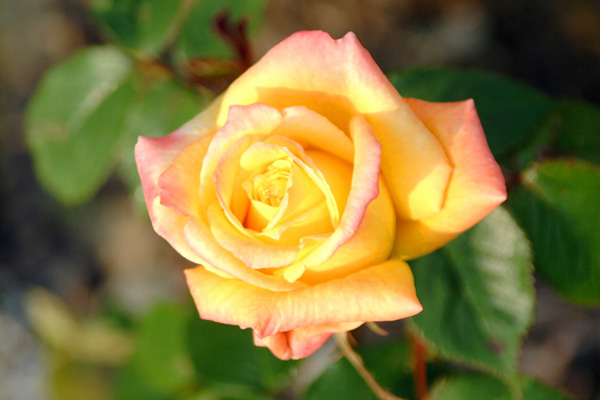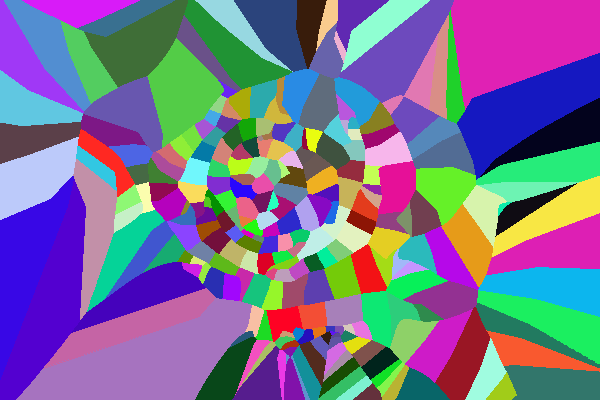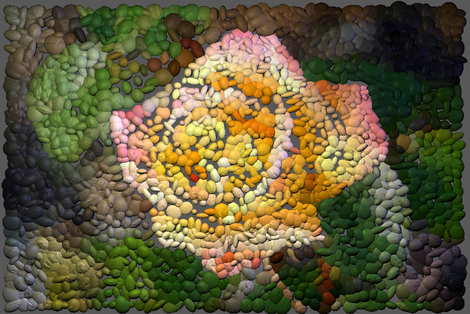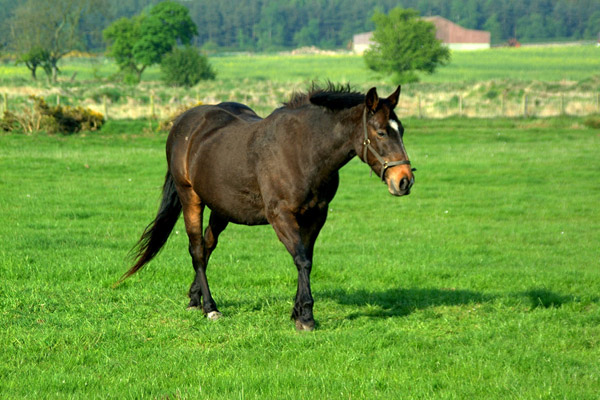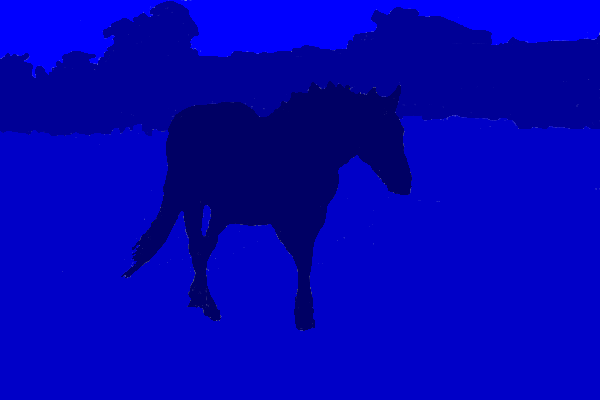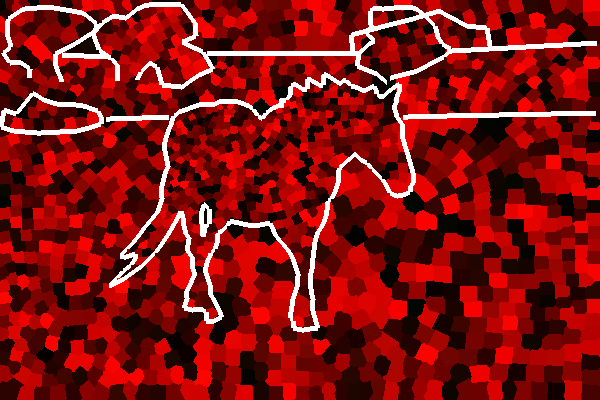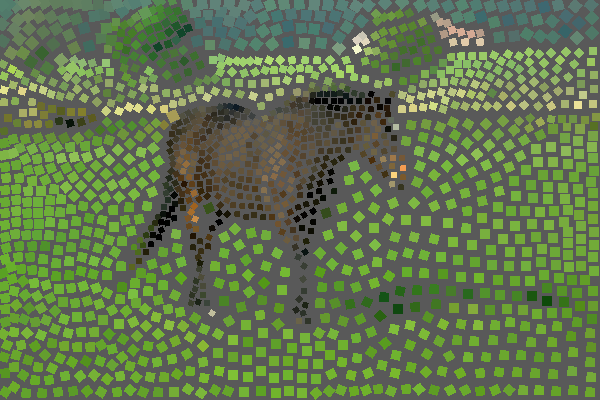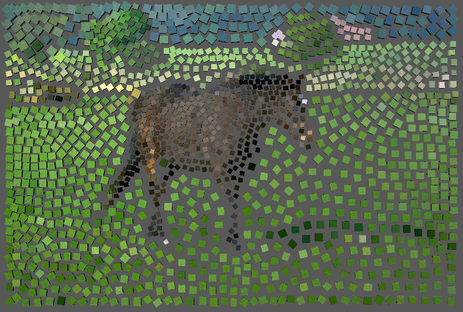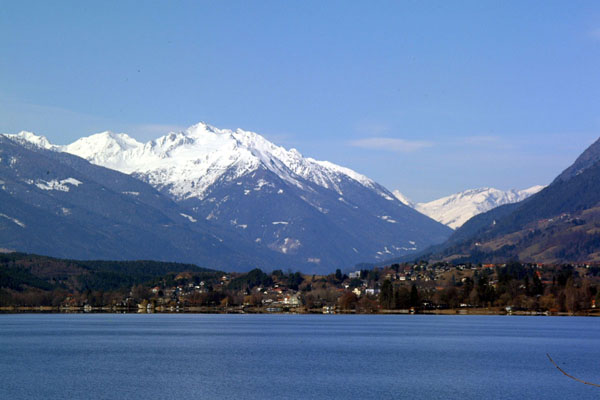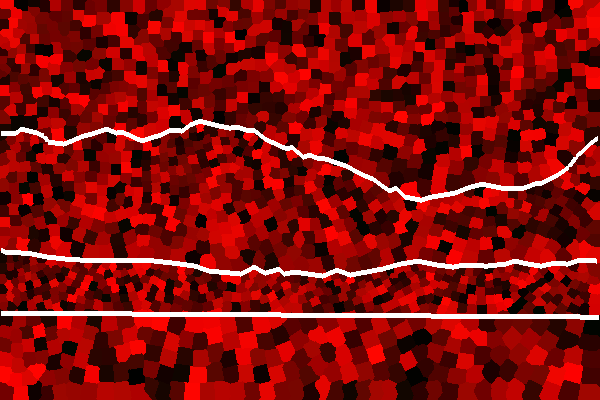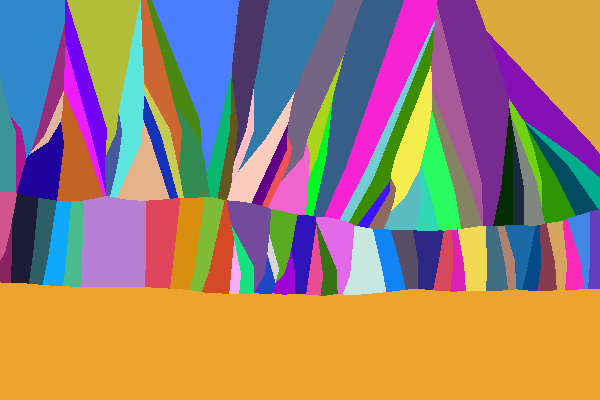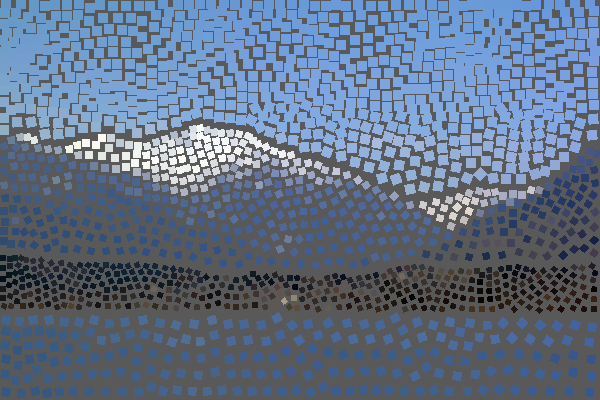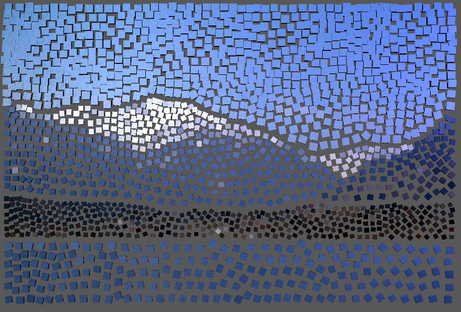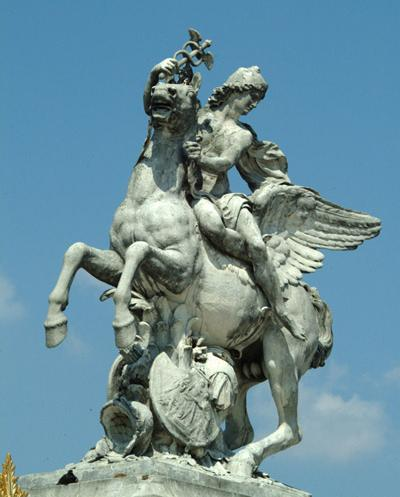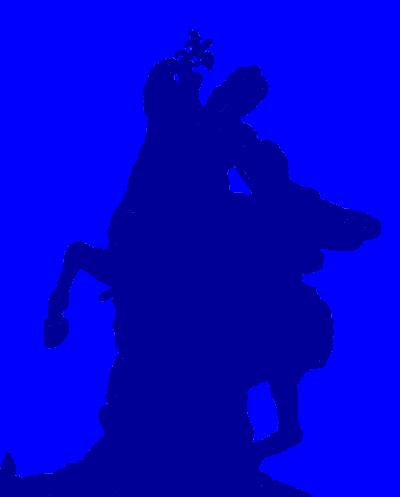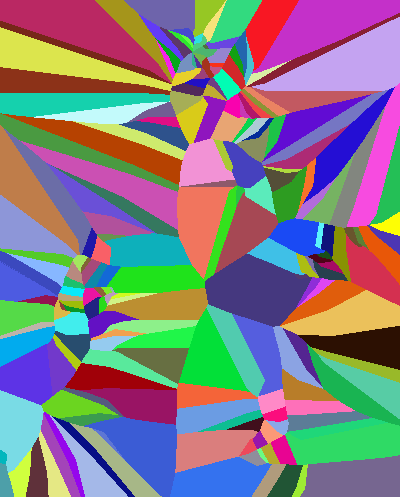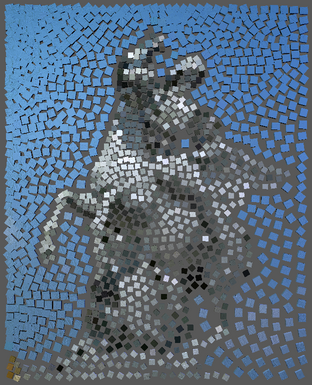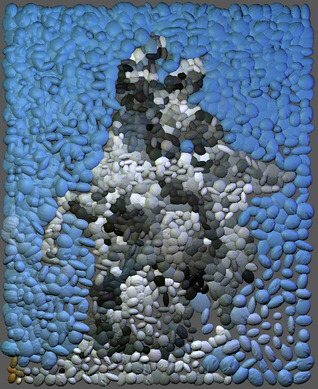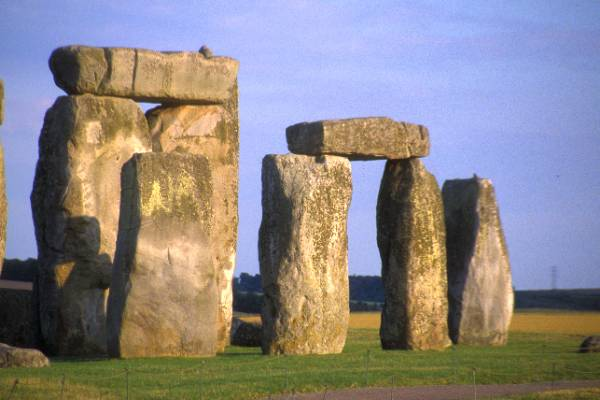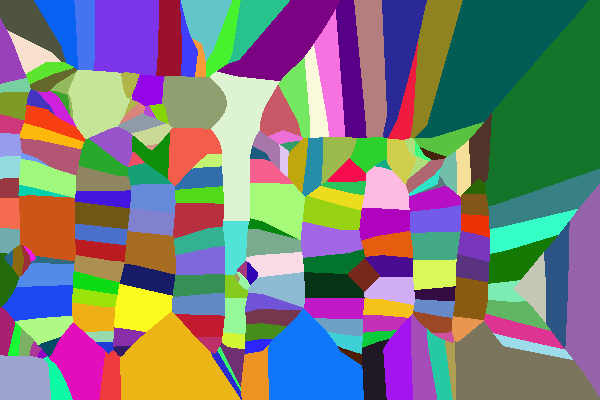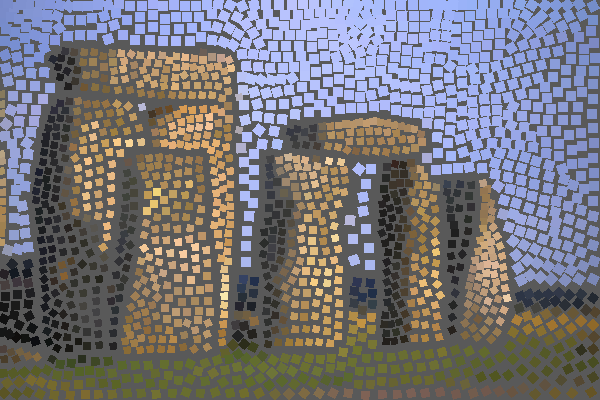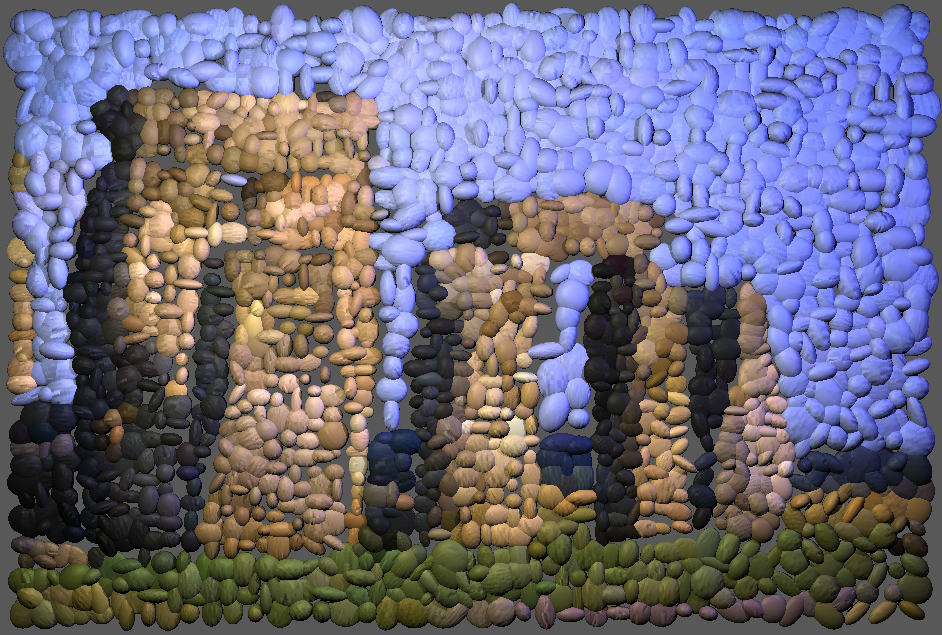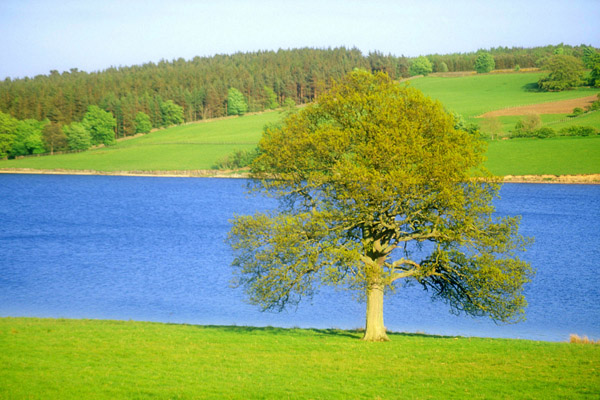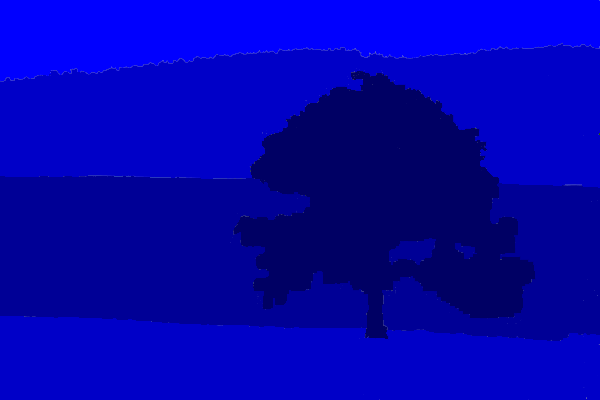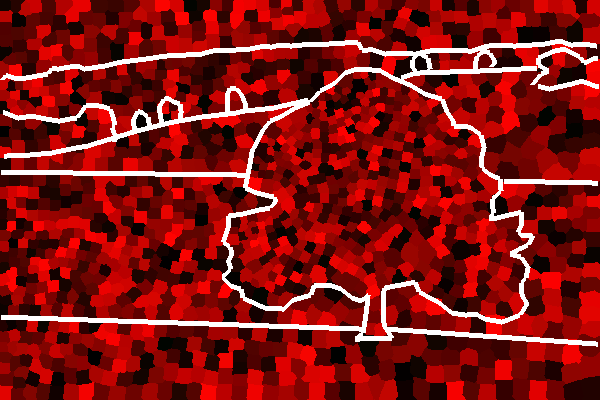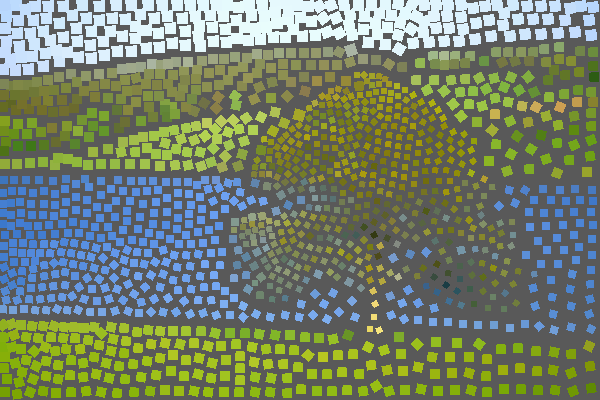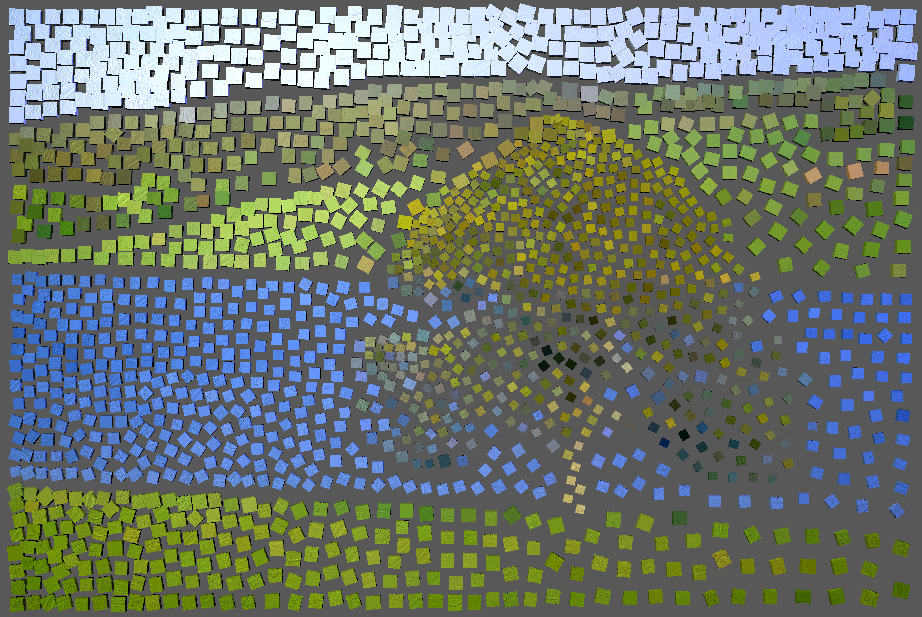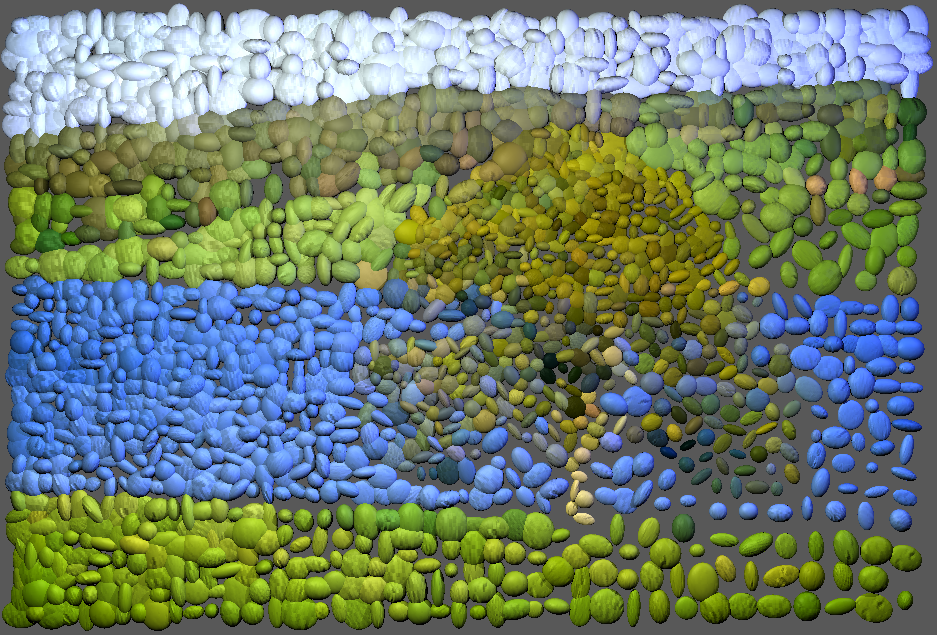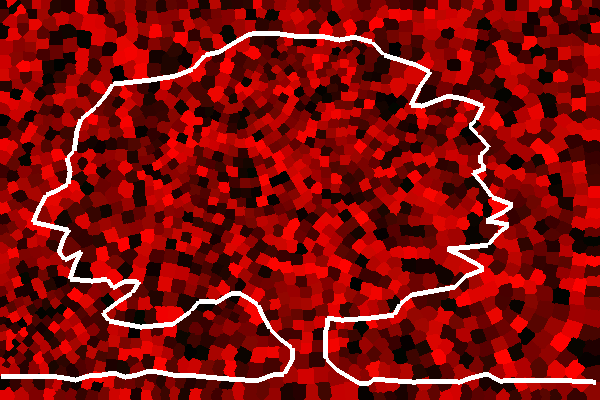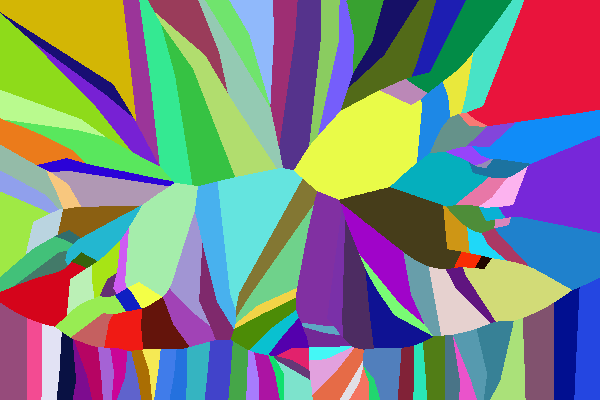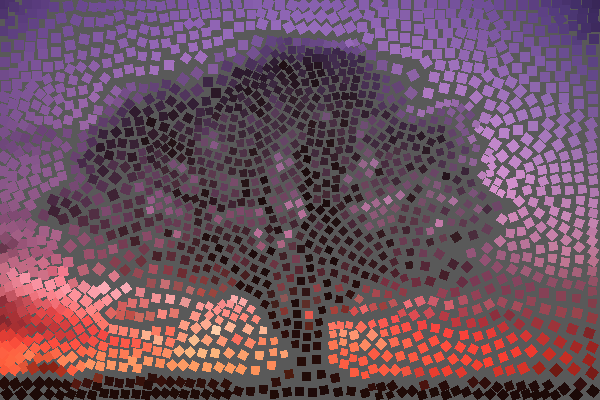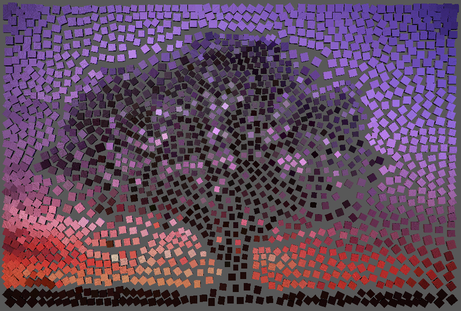# CS798 Project: Simulating Decorative Mosaics

## CGL, University of Waterloo

Decorative mosaic is an interesting art. It is also a research topic in NPR. Unlike stroke based rendering methods, mosaic uses tiny tiles as "stroke". It gives us a different feeling. Because we have done something about stroke, silhouette in assignments, I want to simulate the basic features of mosaic as a new work. Hausner's paper is a good start.

Algorithm:

The basic algorithm includes such steps:
1. Generate a set of points randomly. For each point, place a square pyramid and align it with direction field. To identify the pyramid, I use a method that is similar with the ID image used in assignment2. Each seed point has a ID number. Then the pyramid color is Colour((ID>>16)&0xff, (ID>>8)&0xff, (ID)&0xff). So by reading the color, we can infer which pyramid it belongs to and get other information.
2. Render the pyramids with an orthogonal projection to produce a Voronoi diagram.
3. Use Lloyd's algorithm to compute the centroid of each Voronoi region and update the diagram.
4. Render the tiles based on the computed information.
Approach:
• Direction field
To decide the orientation of tiles, a direction field is used. The direction field is also computed from Voronoi diagram. I implemented it in three steps:
1. Compute generalized Voronoi diagrams along edges using forward discussed method. The edges are defined by the user.
2. Get depth data from z-buffer, and take z-buffer data as source image. Using central differencing to compute the gradient in this image.
3. Rotate the rendering units(pyramids) along the gradient direction.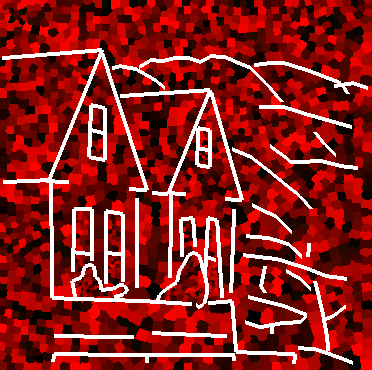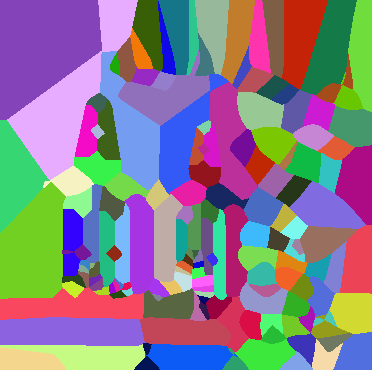Figure1: User defined edge, edge's Voronoi diagram, direction field.
• Edge avoidance
To prevent the tiles straddling the edge, a simple method is used. First, the user draw the thick edge lines with a unique color. I set the width of these lines 5 pixels, and use white color to express edge line. If edge overwrites some part of a tile, from centroid algorithm, we can conclude that the tiles will be moved away from edge. Because there are fewer pixel contribute to the centroid computing. So the centroid will be moved to the position away from the edge. Obviously, it will generate gaps where edge was. The following figures show the procedure.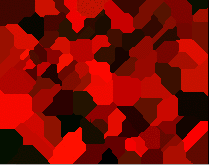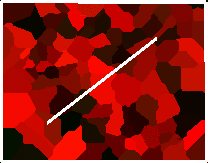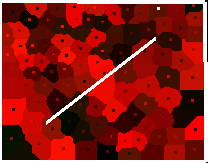Figure2: Edge avoidance. From left to right: initial stage, user defined edge, result after 5 iterations, after 10 iterations, final result after 20 iterations, final tiling.

• Tile rendering
Using the algorithm, we can get the basic information such as position, orientation for each tile. We also can setother attributes. For example, we can get the color of tile by sampling at a point or compute the average region color in this tile. In my program, I use average color. The tile size also can be changed. The normal size is defined by this formula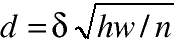. I set the constant delta to 0.8. The h and w are the size of source image and n is the number of seed points. We modify the equation of Manhattan distance so that the tile size will be changed.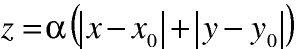. Alpha is a parameter to control the tile size. In my implementation, the user provide a mask image. It defines the alpha value for each pixel. By sampling the mask image, we can create different tiles. The mask image is set with different colors, and each color mapping to a alpha value. In order to avoid conflicting with the color of Voronoi diagram, I set the color of mask image such that they only have blue component. And I defined such mapping:
blue color component: 0xff -- alpha: 1.0
blue color component: 0xc8 -- alpha: 0.8
blue color component: 0x96 -- alpha: 0.6
blue color component: 0x64 -- alpha: 0.4
blue color component: 0x32 -- alpha: 0.2

Example:

The following example shows the full procedure. The input image is a pattern of Yinyang. There are 300 seed points.
Then the user defines the edges and computes according Voronoi diagram. Computing the centroid using Lloyd's method. The final tiling is the result after 20 iterations.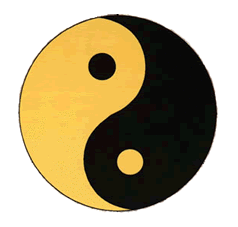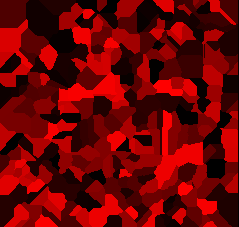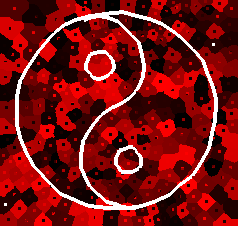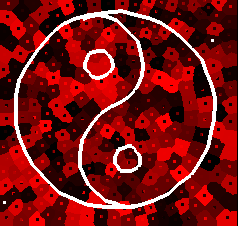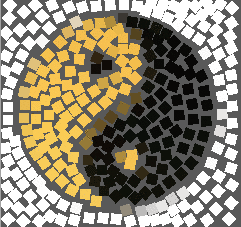Figure3: Procedure of generating a mosaic. From left to right: source image, initial stage, user defined edge, edge's Voronoi diagram, result after 5 iterations, after 20 iterations and final tiling.

Another more complex example is from Hausner's paper. I set 2000 seed points. Then input the edges and mask image. Compute the direction field. Finally generate the tiling after iterating 20 times. Figure4 displays this example.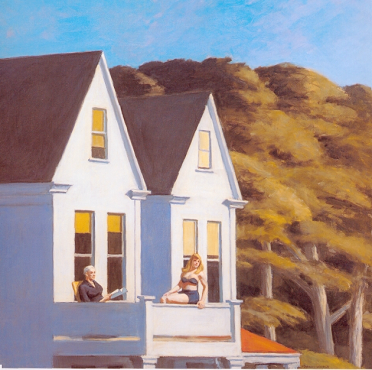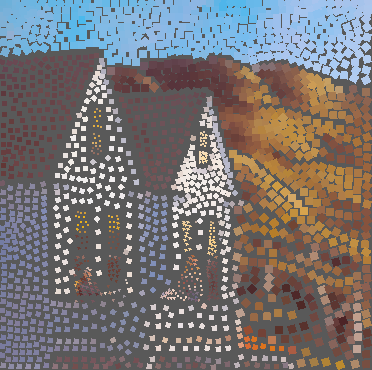Figure4: Another example. Source image, initial stage, mask image, user defined edge, edge's Voronoi diagram, final tiling.

Extensions:

1. I also did some simple extensions. People usually use dark color tile to enforce the edge of shape in moscaics. I simulate it by displaying the edge lines. I also test the result render litter tiles instead of lines. Figure5 shows my result.

1. I find that there are some similar arts, for example, pebble mosaic. Pebble mosaic is using small pebbles to tile patterns on the road or wall. Pebble mosaic is an interesting artistic style. In order to simulate this style, I first generate the normal tiling with the basic algorithm, then tranform the tile from flat square to ellipsoid and using tiny random number to perturb the size. I output the result into python script file and render it using my ray tracer which is created in CS688. To enhance the result, I also use bump mapping in rendering. The bump textures are distilled from real pebble pictures and attribute to pebble tile randomly. I also render the mosaic using cube tile. Figure6 shows my result.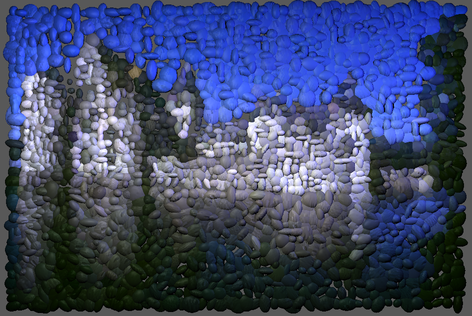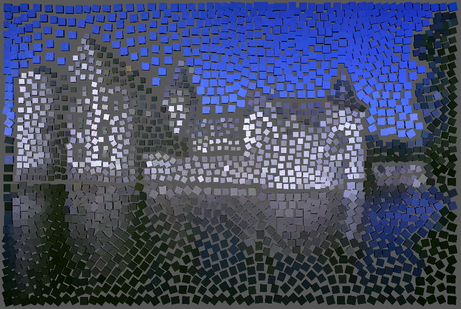Figure6:  Simulated pebble mosaic and cube tile mosaic.

1. Another extension is to simulate different mosaic styles. For example, the opus classicum and opus vermiculatum styles. To simulate the opus vermiculatum style, I just draw some parallel edges. Figure7 shows this example.

Figure7: Simulated opus vermiculatum style

To simulate the opus classicum style, I constructed it with two layers. In the first layer, I used regular pattern to tile the background. In the second layer, I created the mosaic. Finally, I combined the two layers to get the desired style. Figure8 shows this style.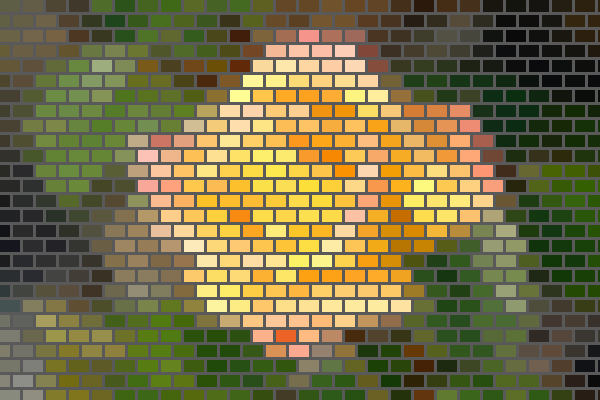Figure8:  Simulated opus classicum style. from left to right: first layer, second layer, combined result.

Bibliography:

Hoff, K., Keyser, J., Lin, M., Manocha, D. and Culver, T. Fast Computation of Generalized Voronoi Diagrams               Using Graphics Hardware. SIGGRAPH 99: 277-286.
Hausner, A. Simulating Decorative Mosaics. SIGGRAPH 2001: 573-580.
Kim, J. and Pellacini, F. Jigsaw Image Mosaics. SIGGRAPH 2002: 657-664.

More results: